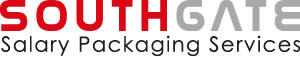FBT

Fringe Benefits Tax (FBT) is the tax payable on all taxable employer-provided benefits or “fringe benefits”. Southgate calculates the FBT on a Novated Lease using the Statutory Formula Method. This calculation is based on the cost price of the vehicle and the number of days in the year the vehicle is packaged (1st April to 31st March).

Car running costs can be salary packaged under a Novated Lease and are exempt from FBT, as the FBT payable only relates to the base cost of the vehicle, not the running costs.

The cost of a novated motor vehicle lease to an employee’s salary package is the total operating cost of the vehicle plus any applicable FBT.

Calculating FBT on a Novated Lease vehicle

There are two steps involved in the calculation of FBT on a Novated Lease, outlined below.

Step 1: Calculate the taxable value of the motor vehicle using the following formula:

Taxable Value = [(A x B) x C ÷ D] – E

Where:
A = base value of the motor vehicle
B = statutory percentage
C = number of days in the FBT year where the vehicle was available for private use by the employee
D = the number of days in the FBT year
E = the recipient contribution (RCM)

The number of kilometres travelled determines which statutory percentage is applied during the calculation of FBT, as displayed in the table below.

 Distance travelled during FBT year STATUTORY PERCENTAGE Leases started before 11 May 2011 Leases started on or after 11 May 2011 0 - 14,999 kms 26% 20% 15,000 - 24,999 kms 20% 20% 25,000 - 40,000 kms 11% 20% More than 40,000 kms 7% 20%

Step 2: Calculate the Fringe Benefits Tax using the following formula

Fringe Benefits Tax = Taxable Value x Gross Up Factor x FBT rate

The ‘gross up factor’ will be 2.0802 when GST is applicable to leasing arrangement (Type 1 benefit).

Example: Calculating FBT using the Statutory Formula Method (without RCM)

Assumptions:

• Vehicle cost base = \$30,000 (excludes on-road costs)
• Annual kilometres = 15,000kms (20% statutory percentage)
• 2.0802 gross-up factor (Type 1 benefit)
• No recipient contribution

Step 1: Calculate the Taxable Value

 Taxable Value = [(A x B) x C ÷ D] – E = [(30,000 x 0.20) x 365/365] – \$0 = \$6,000

Step 2: Calculate the FBT

 Fringe Benefits Tax = Taxable Value x Gross Up Factor x FBT Rate = \$6,000 x 2.0802 x 0.47 = \$5,866.16